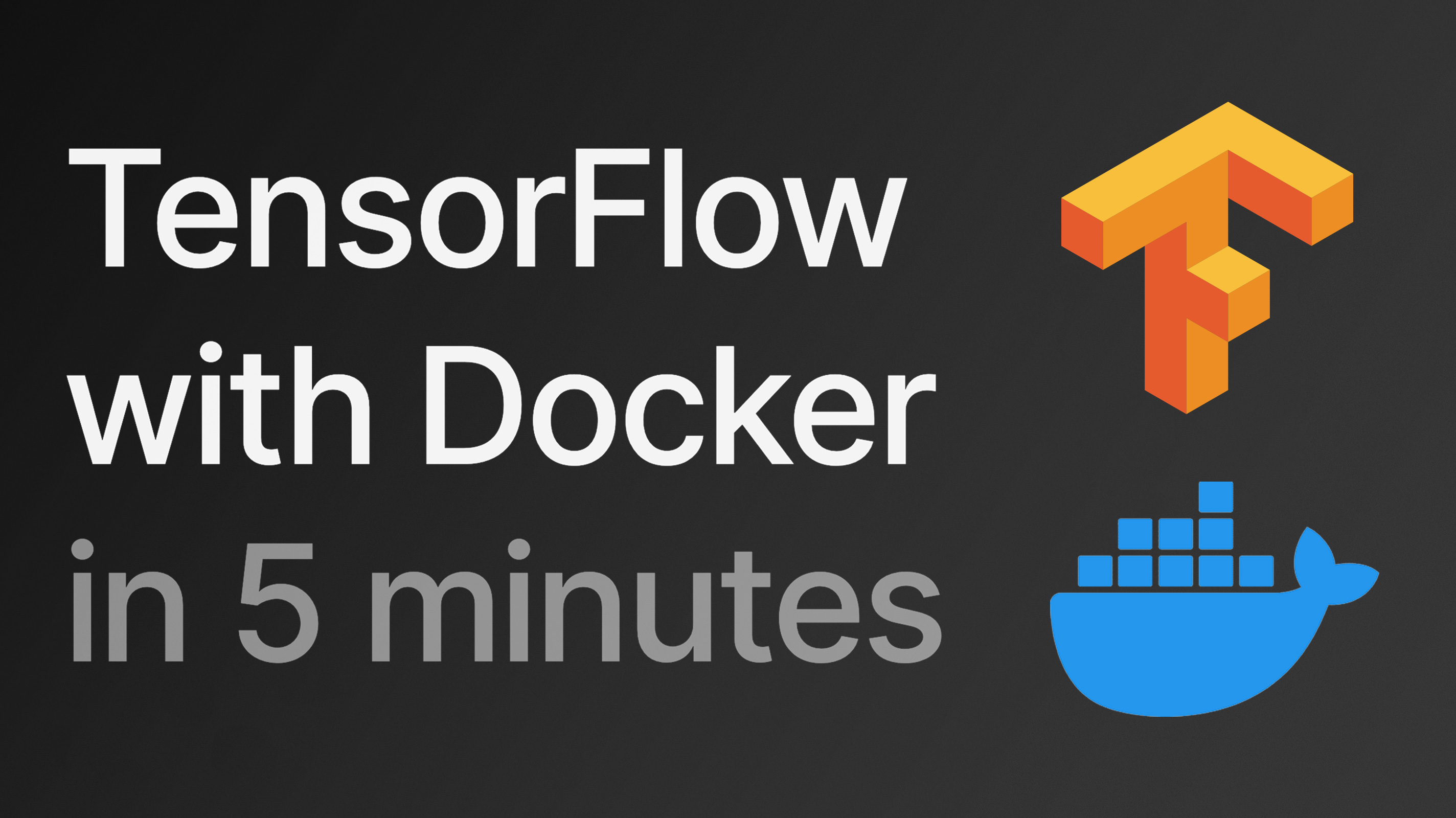# Run TensorFlow on a Docker container

NOVEMBER 3, 2022Here's how to run TensorFlow inside of a Docker container.

``````# Start a Docker container
# with an interactive bash session
docker run -it python:3.9-slim bash

# Install TensorFlow and TensorFlow I/O
pip install tensorflow tensorflow-io

# Run TensorFlow in Python
python -c "import tensorflow as tf;\
print(tf.constant(42) / 2 + 2);\
print(tf.convert_to_tensor([1,2,3]))"
# tf.Tensor(23.0, shape=(), dtype=float64)
# tf.Tensor([1 2 3], shape=(3,), dtype=int32)
``````

This approach is useful when you don't want to install TensorFlow locally or create a Python environment, or simply when it's hard or not possible to install TensorFlow in your local runtime.

Docker makes it quick to execute TensorFlow commands in Python on any machine running Docker.

If you don't need an interactive session and can define your Python code directly, you can use this one-liner.

``````docker run -it python:3.9-slim \
bash "-c" "pip install tensorflow tensorflow-io;\
python -c 'import tensorflow as tf; \
print(tf.constant(42) / 2 + 2); \
print(tf.convert_to_tensor([1,2,3]))'"
# tf.Tensor(23.0, shape=(), dtype=float64)
# tf.Tensor([1 2 3], shape=(3,), dtype=int32)
``````